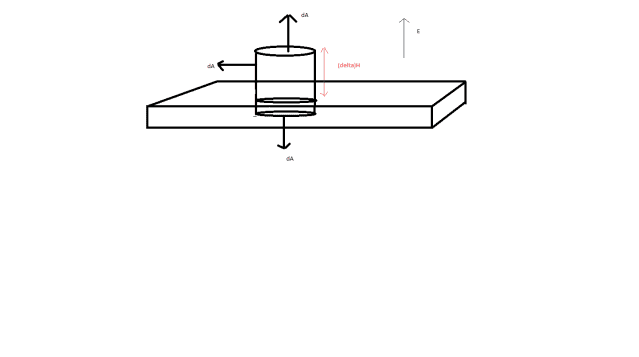# Electric field on a surface

• Sixty3

## Homework Statement

A metallic surface is placed in an electrostatic field. Derive an expression for the electric field on the metal surface.

## Homework Equations

$\oint \underline{E} \cdot d\underline{A}=\dfrac{q_{enc}}{\epsilon_0}$

## The Attempt at a Solution

My initial thought was to set up a cylindrical Gaussian surface, I've tried to show it in the picture attachment.Then the dA facing downwards will be 0 since the electric field within the metal is 0, and the 'side' of the cylinder dA is perpendicular to the electric field and also 0. So the only contribution is the upward facing circular area. Letting Δh→0, it gives the electric field on the surface, EA=q/ε, and q/A=σ the surface charge density. So E=σ/ε.

Is this method correct, I'm not entirely sure to be honest.

Looks a bit like you're overthinking it. All that matters is the total surface area of this cylinder in this case.

So, how could you calculate the total surface area?

Well, you've got two circles at each end of the cylinder, so that surface area would be two times the area of a circle.
$$A_{1} = 2\pi r^2$$

For the second part of calculating the surface area, it would just be the total length of your cylinder times the length, ##l##, of the cylinder.
$$A_{2} = 2\pi r l$$

Total surface area:
$$SA = A_{1}+A_{2}$$

Plugging into Gauss's Law and simplifying yields:
$$E = \frac {2 k_{c} q_{enc}}{r(l+r) }$$

Note that $$k_{c} = \frac {1}{4\pi \epsilon_{0}}$$ so this is how the ##k_{c}## appeared.

The total field is the sum of the external applied field plus the field due to the charge on the surface of the metal. Inside the metal the fields are in opposite direction and cancel. Outside they are in the same direction and add up.

Looks a bit like you're overthinking it. All that matters is the total surface area of this cylinder in this case.

So, how could you calculate the total surface area?

Well, you've got two circles at each end of the cylinder, so that surface area would be two times the area of a circle.
$$A_{1} = 2\pi r^2$$

For the second part of calculating the surface area, it would just be the total length of your cylinder times the length, ##l##, of the cylinder.
$$A_{2} = 2\pi r l$$

Total surface area:
$$SA = A_{1}+A_{2}$$

Plugging into Gauss's Law and simplifying yields:
$$E = \frac {2 k_{c} q_{enc}}{r(l+r) }$$

Note that $$k_{c} = \frac {1}{4\pi \epsilon_{0}}$$ so this is how the ##k_{c}## appeared.

That solution is not correct. The side area does not contribute because the field is parallel to the surface.

Imagine a parallel plate capacitor with potential V across the plates, and you slide a plate inbetween the plates. What is the E field before & after sliding the plate?

(You can use 5 Gaussian surfaces to derive 6 equations in 6 unknowns; the last equation has to do with the voltage V).